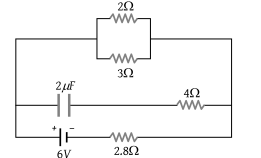In the figure shown, the capacity of the condenser C is $2\text{\hspace{0.17em}}\mu F$. The current in 2 Ω resistor is(1) 9 A

(2) 0.9 A

(3) $\frac{1}{9}A$

(4) $\frac{1}{0.9}A$